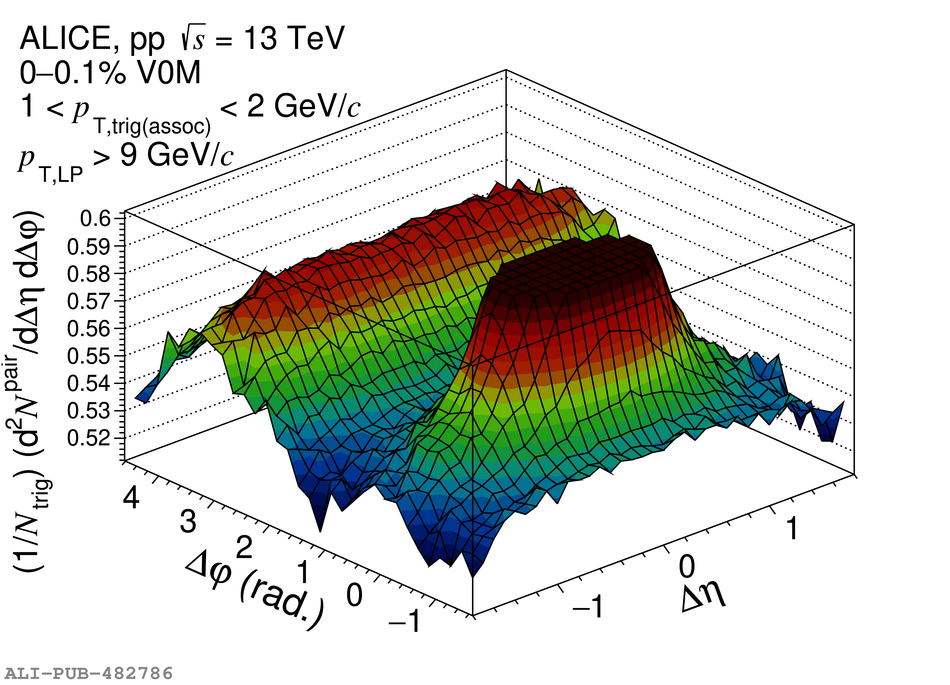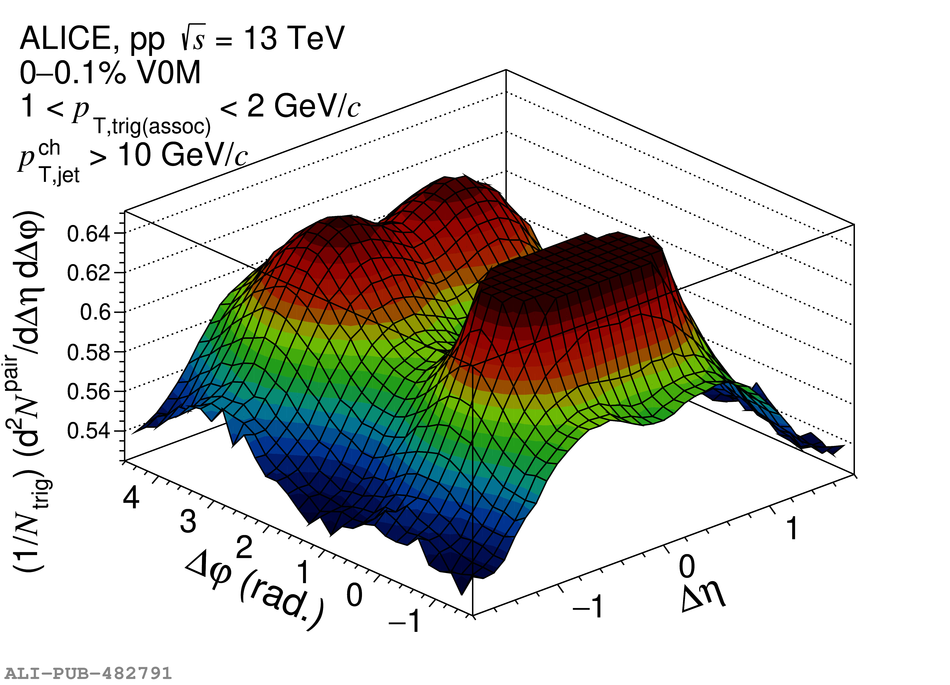# Figure 4

 < p> Two-dimensional correlation functions as a function of $\Delta\eta$ and $\Delta\varphi$ in high-multiplicity events including a selection on the event-scale. The interval of $\pttrig$ and $\ptassoc$ is 1~~9~GeV/$c$ leading track. Right: HM events with a $\ptjet>$~10~GeV/$c$.< /p>